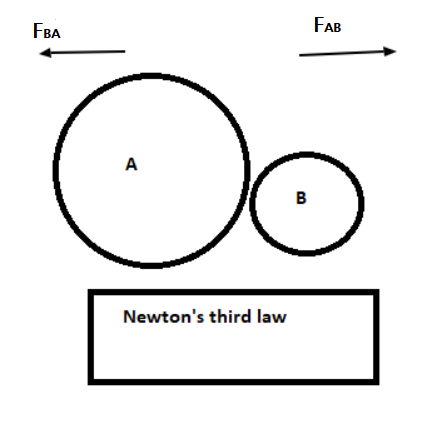Home/Class 9/Physics/

Describe Newton’s third law of motion.Speed
00:00
07:10## QuestionPhysicsClass 9

Describe Newton’s third law of motion.

.
Newton’s third law of motion states that 'to every action, there is an equal and opposite reaction'. When an object, say $$A$$, exert a force on another object, say $$B$$, then $$B$$ also exerts a force on the $$A$$. These two forces are always equal in magnitude but opposite in direction.As shown in the above figure, if $${F}_{AB}$$ be the force exerted by body $$A$$ on $$B$$ and $${F}_{BA}$$ is the force exerted by $$B$$ on $$A$$,
then according to Newton's third law
$${F}_{BA}=- {F}_{AB}$$
Force on $$A$$ by $$B = -$$ Force on $$B$$ by $$A$$
Reaction $$= \ –$$ Action
This law clarifies that a single force can never exist and that the forces always exist in pairs. The two opposing forces are known as action and reaction. The forces of action and reaction always act on two different bodies simultaneously.